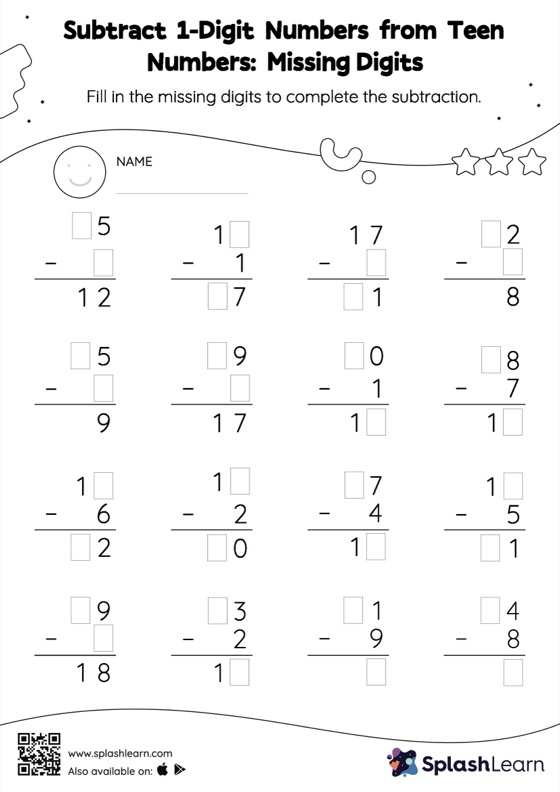# Subtract 1-Digit Numbers from Teen Numbers: Missing Digits Worksheet

Home > Subtract 1-Digit Numbers from Teen Numbers: Missing DigitsStudents use the count back strategy or the relationship between addition and subtraction to find the missing number in this worksheet. However, they do not need to regroup numbers to get to the answer in subtract 1-digit numbers from teen numbers worksheet. As the worksheet uses the column method, it is helpful in getting students toward higher accuracy, especially with bigger numbers and in scenarios where regrouping is required.